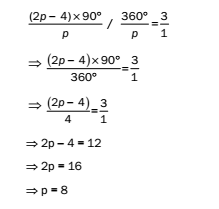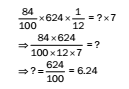# RRB ALP 2018 Practice Test Papers | Arithmetic Questions (Day-24)

Dear Aspirants, Here we have given the Important RRB ALP & Technicians Exam 2018 Practice Test Papers. Candidates those who are preparing for RRB ALP 2018 can practice these Arithmetic Questions to get more confidence to Crack RRB 2018 Examination.

[WpProQuiz 1879]

Click “Start Quiz” to attend these Questions and view Explanation

1. If the volume of a sphere is numerically equal to its surface area, then its diameter is
1. 4 unit
2. 2 unit
3. 3 unit
4. 6 unit
1. If the sum of LCM and HCF of two numbers is 66 and their difference is 44, what is the product of the numbers?
1. 2904
2. 726
3. 1210
4. 605
1. Three cloths of length 7 m, 7.5 m and 9.5 m, respectively have to be cut into the same length pieces of the maximum size. What will be the total number of pieces?
1. 24
2. 48
3. 96
4. 120
1. If one-fourth of one-fifth of a number is 4, then find its three-fourth.
1. 24
2. 36
3. 48
4. 60
1. A number consists of 3 digits whose sum is 12. The middle digit is twice the sum of the first and the third digit and the number will be increased by 198 if its digits are reversed. Find the number.
1. 191
2. 187
3. 179
4. 183
1. The ratio of each interior angle to each exterior angle of a regular polygon is 3: 1. The number of sides of the polygon is
1. 7
2. 9
3. 8
4. 6
1. The value of the question mark (?) in the following expression is 84% of 624 ÷ 12 =? × 7
1. 63
2. 6.24
3. 6.34
4. 6.56
1. If a2bc = a4b = 2025, what is the value of 2c + 2?
1. 15
2. 20
3. 25
4. 30
1. A student scores 55% marks in 8 papers of 100 marks each. He scores 15% of his total marks in English. How much does he score in English?
1. 55
2. 66
3. 77
4. 44
1. A can work at a speed thrice faster than B. Together, they can finish a work in 6 days. How many days will B alone take to finish the work?
1. 20
2. 15
3. 10
4. 24

Let radius of sphere = r unit

Then, 4/3 (πr3) = 4πr2⇒ r = 3 unit

So diameter = 2r = 6 unit

LCM + HCF = 66 ……(1)

LCM – HCF = 44 ……(2)

By adding (1) and (2), we get:

2 × LCM = 110

LCM = 55

Putting LCM = 55 in (1), we get: 55 + HCF = 66

HCF = 11

Product = 55 × 11 = 605

7 m = 700 cm

7.5 m = 750 cm

9.5 m = 950 cm

HCF of 700 cm, 750 cm and 950 cm = 50 cm

Number of pieces 700/50+ 750/50+ 950/50= 14 +15 +19= 48

1 /4 x1/ 5 × X = 4

X = 80

Therefore three-fourth of 80 = 60

Let the number of three digits be abc = 100a + 10b + c

According to question, a + b + c = 12

b = 2(a + c) and 100c + 10b + a – 100a – 10b – c = 198

c – a = 2

Therefore, a = 1, b = 8 and c = 3 and hence the required number is 183.

Let the number of sides of polygon = p

According to the question,8. Answer: (b)

a2bc = a4b

=>c = a2

2025 = 3 × 3 × 5 × 5 × 3× 3 = 34 × 25

By comparing with a4b, we get: a = 3; b = 25 and c = a2 = 9

Therefore, 2c + 2 = 2 × 9 + 2 = 20.

Total marks = 8 × 100 = 800

Marks scored by the student = 55% of 800 = 440

Marks scored in English = 15% of 440 = 10% of 440 + 5% of 440 = 44 + 22 = 66

Let us assume that A can complete the work in x days.

So, B can complete the work in 3x days.

In one day, they can together complete(  1/ x+1/ 3x )of the work = 4/ 3x of the work

∴ 4/ 3x = 1/ 6  =>x = 8

∴ Time taken by B to finish the work by working alone = 3 × 8 = 24 days

RRB ALP 2018 Practice Test Papers | Arithmetic Questions (Day-1)

RRB ALP 2018 Practice Test Papers | Arithmetic Questions (Day-2)

RRB ALP 2018 Practice Test Papers | Arithmetic Questions (Day-3)

RRB ALP 2018 Practice Test Papers | Arithmetic Questions (Day-4)

RRB ALP 2018 Practice Test Papers | Arithmetic Questions (Day-5)

RRB ALP 2018 Practice Test Papers | Arithmetic Questions (Day-6)

RRB ALP 2018 Practice Test Papers | Arithmetic Questions (Day-7)

RRB ALP 2018 Practice Test Papers | Arithmetic Questions (Day-8)

RRB ALP 2018 Practice Test Papers | Arithmetic Questions (Day-9)

RRB ALP 2018 Practice Test Papers | Arithmetic Questions (Day-10)

RRB ALP 2018 Practice Test Papers | Arithmetic Questions (Day-11)

RRB ALP 2018 Practice Test Papers | Arithmetic Questions (Day-12)

RRB ALP 2018 Practice Test Papers | Arithmetic Questions (Day-13)

RRB ALP 2018 Practice Test Papers | Arithmetic Questions (Day-14)

RRB ALP 2018 Practice Test Papers | Arithmetic Questions (Day-15)

RRB ALP 2018 Practice Test Papers | Arithmetic Questions (Day-16)

RRB ALP 2018 Practice Test Papers | Arithmetic Questions (Day-17)

RRB ALP 2018 Practice Test Papers | Arithmetic Questions (Day-18)

RRB ALP 2018 Practice Test Papers | Arithmetic Questions (Day-19)

RRB ALP 2018 Practice Test Papers | Arithmetic Questions (Day-20)

RRB ALP 2018 Practice Test Papers | Arithmetic Questions (Day-21)

RRB ALP 2018 Practice Test Papers | Arithmetic Questions (Day-22)

RRB ALP 2018 Practice Test Papers | Arithmetic Questions (Day-23)